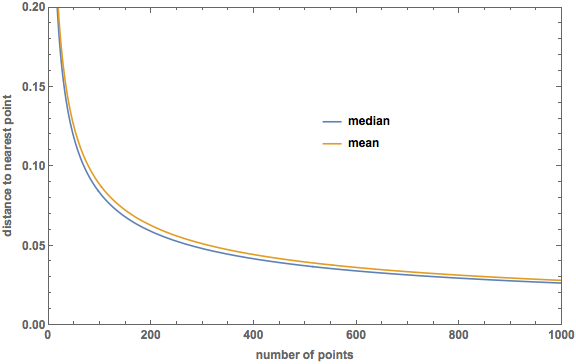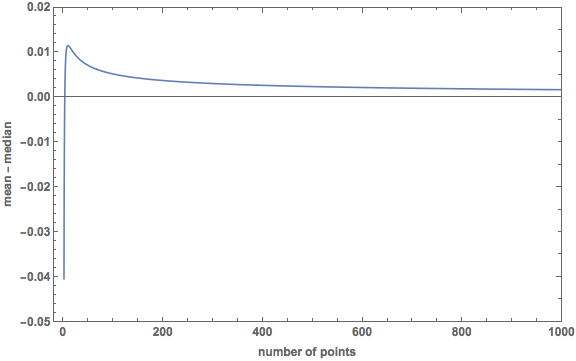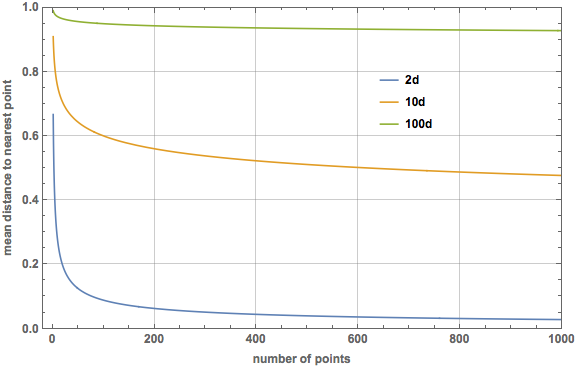# Mean Distances in p-dimensions

In my last post, I calculated the mean distance to the nearest point to the origin among $$N$$ points taken from a uniform distribution on $$[-1, 1]$$. This turned out to be $$r = 1/(N+1)$$, which is close to but greater than the median distance $$m = 1 - (1/2)^{1/N}$$.

In this post, I want to generalize the calculation of the mean to a $$p$$-dimensional uniform distribution over the unit ball. To start things off, let’s look at the specific case $$p = 2$$ (a circle). It is often easier to see a generalization after performing the calculation in a few easy (low) dimensional cases.

Following the same form of reasoning as in the previous post, we want to calculate $\text{Pr}(\text{ closest point is at } r) = \text{Pr}(\text{ all other points are } > r) \times \text{Pr}(\text{ one point is at } r) .$ The probability that all other points $$(N -1)$$ are at distances greater than $$r$$ is $$(1-r^2)^{N-1}$$. The probability that one point is at a distance $$r$$ is the ratio of the infinitesimal area at $$r$$ to the entire area of the unit circle: $\frac{2 \pi r\, \text{d}r}{\pi} = 2 r\, \text{d}r.$ We also have to mutiply by an overall factor of $$N$$ to account for the $$N$$ different ways of labelling the closest point. Putting everything together: $\text{Pr}(\text{ closest point is at } r) = 2 r N (1- r^2)^{N-1} \text{d} r,$ and to get the mean value of $$r$$ we multiply by $$r$$ and integrate: $\text{E}(r) = \int_0^1 2 r^2 N (1-r^2)^{N-1} \text{d}r = N \sqrt{\frac{\pi}{4}} \frac{\Gamma(N)}{\Gamma(N + \frac{3}{2})}.$ I used Mathematica to carry out the integral and verified the answer with Gradshteyn and Ryzhik, 3.251, number 1 (page 324 in the 7th edition). In Gradshteyn and Ryzhik, the integral is given in terms of the beta function (Euler integral of the first kind): $B(x, y) = \frac{\Gamma(x)\Gamma(y)}{\Gamma(x+y)}.$ I don’t see a nice simplified form, and Mathematica doesn’t seem to be able to find one.

Here is a plot made in Mathematica comparing the median distance to the nearest point ($$m = (1 - (1/2)^{1/N})^{1/2}$$) to the mean:In 2 dimensions, the mean is not always greater than the median. Here is a plot of the difference:The mean starts out smaller than the median, and they are equal at $$N = 2.6237$$. The peak in the plot (when the mean is most greater than the median), occurs at $$N = 0.9807$$ and the value of the difference here is $$0.0115$$.

To generalize this to $$p$$-dimensions, the probability that $$(N-1)$$ points are at a distance $$> r$$ is now $$(1 - r^p)^{N-1}$$ (look back at my post on the median distance for an explanation). The probability that one point is at $$r$$ is $\frac{ A_{p-1}(r) \text{d}r }{V_p(1)},$ where $$A_p(r)$$ is the surface area of the $$p$$-sphere of radius $$r$$ (recall the the $$p$$-sphere is the boundary of the $$(p+1)$$-ball, so the 1-sphere is what we ordinarily call a circle and has $$A_1 = 2 \pi r$$). Through some relations between area and volume (check the Wikipedia page if you are interested), it is possible to reduce this to the simple form $\frac{ A_{p-1}(r) \text{d}r }{V_p(1)} = p r^{p-1} \text{d}r.$ Again we have to multiply by the combinatorical factor $$N$$, and the expectation integral in $$p$$-dimensions becomes $\text{E}(r) = \int_0^1 N p r^p (1-r^p)^{N-1} \text{d} r = \frac{N}{p} \frac{\Gamma(N)\Gamma(\frac{1}{p})}{\Gamma(1 + N + \frac{1}{p})}.$ The integral was done in Mathematica and verified using the Gradshteyn and Ryzhik reference above. Here is a plot of the mean distance to the nearest point for dimensions 2, 10, and 100. On this scale, the median lines are barely visually distinguishable from the mean lines, so I did not include them.So the curse of dimensionality is evident using the mean instead of the median, just with a much more difficult (but fun) calculation!##### Landon Lehman
###### Data Scientist

My research interests include data science, statistics, physics, and applied math.